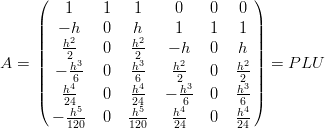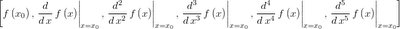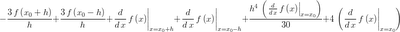## Friday, May 1, 2009

### Deriving Compact Differences with Maxima

I wrote a while back about using Maxima to derive asymmetric complex step finite difference expressions for derivatives. The basic approach was sound, but it didn't really use much of the power of the built-in symbolic computation capability. This post will explore deriving compact difference relations with Maxima and avoid all the for-loops by using Maxima's built-in knowledge of Taylor series and Linear Algebra.

First we'll use the taylor() function to define truncated Taylor series expressions for the function and it's first derivative. Then we'll us the ev() function to evaluate the expressions with different values of x: x0, x0+h, and x0-h. Where h is the step size.

f_taylor : taylor(f(x),x,x0,5);
fp_taylor : taylor('diff(f(x),x),x,x0,4);

rhs_list : [at(f(x),x=x0),
'at('diff(f(x),x), x=x0),
'at('diff(f(x),x,2), x=x0),
'at('diff(f(x),x,3), x=x0),
'at('diff(f(x),x,4), x=x0),
'at('diff(f(x),x,5), x=x0)
];

f_coefs : submatrix(factor(augcoefmatrix([f_taylor], rhs_list)), 7);
fp_coefs : submatrix(factor(augcoefmatrix([fp_taylor], rhs_list)), 7);

Using the augcoefmatrix() function is an easy way to extract the coefficients of the various derivatives in the Taylor series. Coefficients of the function expansion:Coefficients in the first derivative expansion:Now we need to pack the coefficients for each point into a matrix.

/* add the columns for the points with function values: */

/* add the columns for the points with function derivative values: */

The matrix A represents the linear system we need to solve to find the coefficients of the function value and its derivative to use in our finite difference approximation.Since we might want to solve for more than one expression, it's a good idea to factor A rather than just inverting it. The matrix should look familiar if you've used a Taylor Table before.

/* we need to invert A to get finite difference formulas so factor it: */
M : lu_factor(A);
[P,L,U] : get_lu_factors(M);Now it is a straight forward matter to generate a right-hand-side vector for our linear system and solve for the unknown coefficients. The numerical rhs-vector represents the derivatives of the function.Putting a one in the appropriate position of the vector gives the corresponding finite difference expression for that derivative.

/* right hand side vector for getting the first derivative: */
b_fp : matrix(,,,,,);
/* coefficients for the compact difference formula: */
a_fp : fullratsimp(lu_backsub(M, b_fp));
/* values vector (function and derivative at the points): */
vv : matrix([at(f(x),x=x0-h),
at(f(x),x=x0),
at(f(x),x=x0+h),
'at('diff(f(x),x),x=x0-h),
'at('diff(f(x),x),x=x0),
'at('diff(f(x),x),x=x0+h)
]);
/* compact difference formula for the first derivative: */
cd_fp : vv.a_fp;
cd_fp_reduced : expand((h**4) * (cd_fp) / 30);

You might notice the extra 1 in the 6th place of the right-hand-side vector, it's there so we don't get the trivial solution of using the value for the derivative at x=x0 for our estimate of the value of the derivative (we obviously don't know it yet). This results in the following difference expression.Which can be multiplied by an arbitrary constant to achieve a more computation ready form.The h**4 term can be neglected, resulting in the well-known fourth order compact (implicit) expression for the first derivative.

Here's the Maxima batch file used for this.